Author: Oscar Cronquist Article last updated on March 22, 2019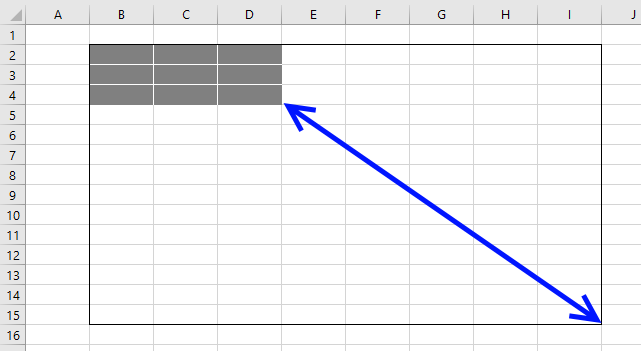In this blog post, I will demonstrate some VBA copying techniques that may be useful if you don't know the exact size of the cell range.

I will also at the very end explain a better solution that is far more easy to programmatically manipulate than a regular cell range.

How do I copy a cell range using VBA?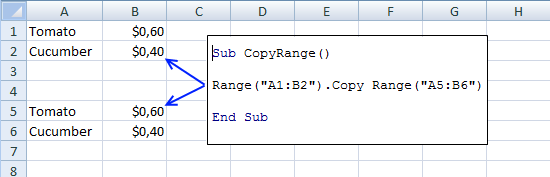This macro copies cell range A1:B2 to A5:B6 in the active sheet. You can make the code even shorter than shown in the image above: Range("A1:B2").Copy Range("A5").

This technique is not dynamic because the ranges are hardcoded in the macro. It will also not only copy values but also cell formatting.

```'Name macro
Sub CopyRange()

'Copy cell range A1:B2 and paste to cell A5
Range("A1:B2").Copy Range("A5")

End Sub
```

The following macro pastes only values not cell formatting.

```'Name macro
Sub CopyRange()

'Copy cell range A1:B2
Range("A1:B2").Copy

'Paste values to cell A5
Range("A5").PasteSpecial xlPasteValues

End Sub
```

Microsoft Excel reference: Worksheet Range Property

How do I copy a dynamic cell range using VBA?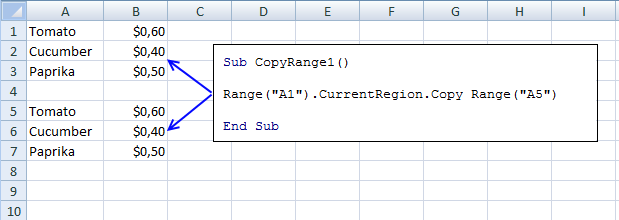A dynamic cell range is a cell range that may change from time to time, you don't know exactly the size.

The current region is a cell range surrounded by blank rows and columns, in other words, non-contiguous or non-adjacent cell ranges

This is equivalent to the shortcut keys Ctrl + A. This works very well if the cell range doesn't have blank rows or columns.

```'Name macro
Sub CopyRange1()

'Use cell A1 to copy it and all adjacent values to cell A5
Range("A1").CurrentRegion.Copy Range("A5")

End Sub
```

Microsoft Excel reference: Range.CurrentRegion Property

How do I copy a dynamic cell range containing blank rows using VBA?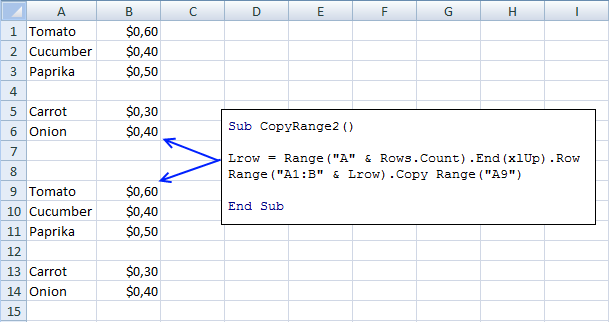The image above shows a blank row making the cell range non-contiguous or non-adjacent. In order to get the entire range Excel starts at the last cell in column A and looks for the first non-empty value upwards.

The row number is then used to build the cell range you want to copy.

```'Name macro
Sub CopyRange2()

'Save row number of last non-empty cell to variable Lrow
Lrow = Range("A" & Rows.Count).End(xlUp).Row

'Copy cell range A1 to last row of non-empty cell and append it to column B then paste to cell A9
Range("A1:B" & Lrow).Copy Range("A9")

End Sub
```

Microsoft Excel reference: End Property

Microsoft Excel reference: Worksheet Rows Property

How do I copy a dynamic cell range containing blank rows and blank cells using VBA?

This example demonstrates a scenario where a cell range (A1:B6) has a blank row (4) and a blank cell (A6), you have to check both columns in order to identify the row of the last non-empty value.

The MAX function returns the maximum row number from these two columns.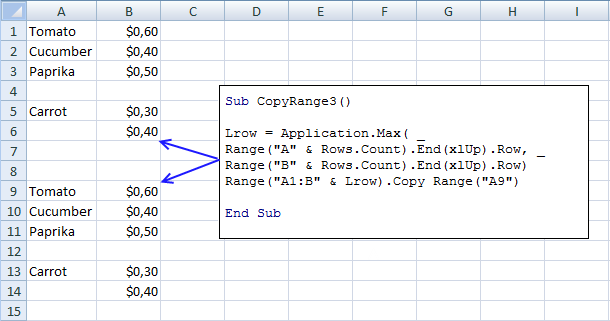```'Name macro
Sub CopyRange3()

'Save largest number of last non-empty row based on column A and B to variable Lrow
Lrow = Application.Max( _
Range("A" & Rows.Count).End(xlUp).Row, _
Range("B" & Rows.Count).End(xlUp).Row)

'Use variable Lrow to build a cell reference then copy cell range and paste to cell A9
Range("A1:B" & Lrow).Copy Range("A9")

End Sub
```

Microsoft Excel reference: End Property

Excel defined Table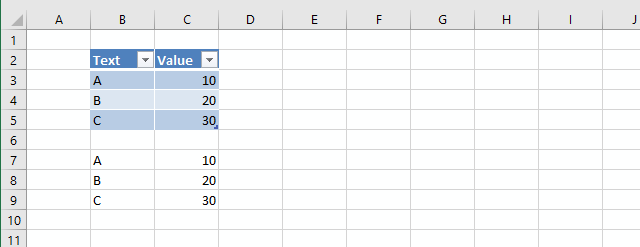I highly recommend you use an Excel defined Table, it is dynamic by design. This way you will save a lot of time and effort to create code that will handle all possible scenarios.

```'Name macro
Sub CopyTable()

'Copy Excel defined Table Table1 located active worksheet
Range("Table1").Copy

'Paste only values to cell B7
Range("B7").PasteSpecial xlPasteValues

End Sub
```

The above macro copies Table1 and pastes only values and not formatting to cell B7, you can see the result in the image above.